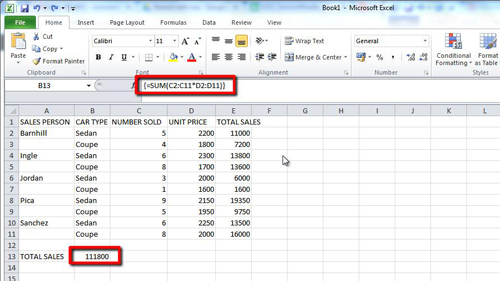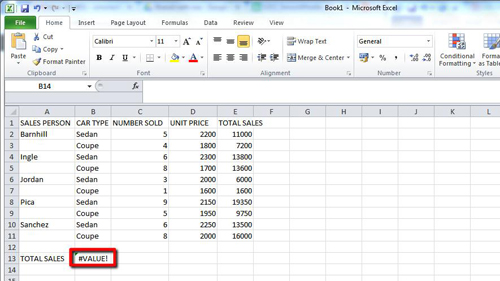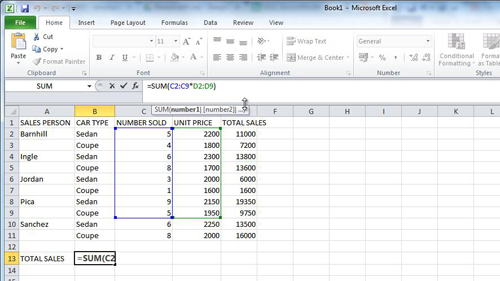## How to Edit Excel 2010 Array

In this tutorial you will learn how to edit excel array formulas which is a bit different from editing standard formulas in Excel.

Step # 1 – Identifying the Array Formula

Open the sheet in Excel that has the array formula in it. Here the array formula is located in cell “B13”. When we click on this cell we can see in the formula bar that it is an array formula as it has braces ({}) around the formula.Step # 2 – Problem when Editing in the Normal Way

Normally to edit the formula you would just make the changes in the formula box, hit return, and the process is complete. As you can see however when we try to change the formula here the braces disappear and the formula returns an error.Step # 3 – Preserving the Array

The reason for this error is that simply pressing return will lose the array feature of the formula. To preserve this, when you have edited the formula you need to press “Control”, “Shift” and “Return” on the keyboard at the same time. Here we will extend the range of the array, press “Control, “Shift” and “Return” and the formula will adjust the total accordingly, and that is how to edit excel array formulas.